# Magnetic Field Orientations in Star Formation

##### Posted on Jan 12, 2022
$\def\MCF{\mathrm{MCF}} \def\pixel{\mathrm{pixel}} \def\cm{\mathrm{cm}} \def\mag{\mathrm{mag}} \def\trs{\mathrm{trs}} \def\small{\mathrm{small}} \def\large{\mathrm{large}}$

Understanding star formation rates (SFRs) is a central goal of modern star formation models, which mainly involve gravity, turbulence and, in some cases, magnetic fields (B-fields).

However, a connection between B-fields and SFRs has never been observed.

A comparison between the surveys of SFRs and a study of cloud-field alignment shows consistently lower SFRs per solar mass for clouds almost perpendicular regulator of SFRs.

The perpendicular alignment possesses a significantly higher magnetic flux than the parallel alignment and thus a stronger support of the gas against self-gravity. This results in overall lower masses of the fragmented components, which are in agreement with lower SFRs.

Clouds with a similar mass and age can have different SFRs, as shown in the following figure.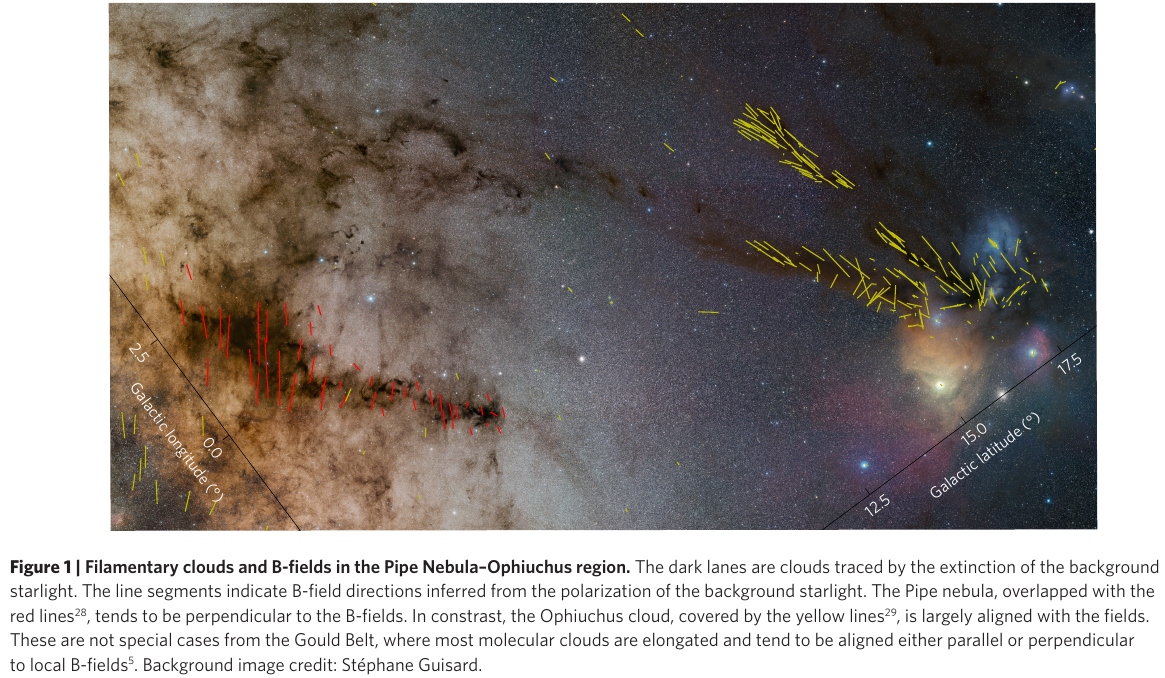Figure 2 correlates the SFRmass$^{-1}$ with the cloud-field angles and shows that large-angle clouds hava consistently lower values of SFRmass$^{-1}$. Perform the permutation tests and Spearman rank correlation tests to determine the significance of this trend.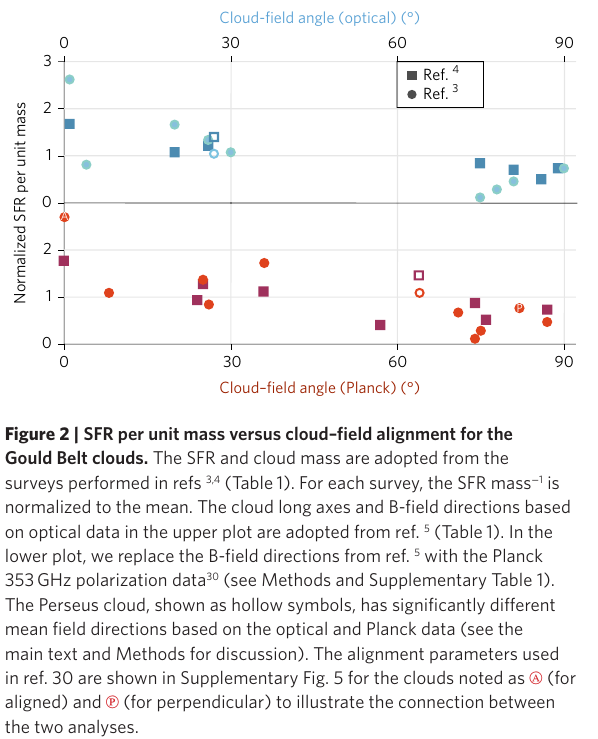The dynamical importance of magnetic fields in molecular clouds has been increasingly recognized, as observational evidence has accumulated. However, how a magnetic field affect star formation is still unclear.

Typical star formation models still treat a magnetic fields as an isotropic pressure, ignoring the fundamental property of dynamically important magnetic fields: their directions.

Their previous work: which demonstrate how the mean magnetic field orientation relative to the global cloud elongation can affect cloud fragmentation.

The paper shows that the mass cumulative function (MCF) of a cloud is also regulated by the field orientation.

• A cloud elongated closer to the field direction tends to have a shallower MCF

The authors have conducted a series of studies on the connections between molecular cloud fragmentation and cloud-field offset (i.e., the misalignment between the ambient magnetic field direction and the cloud elongation).

• Li et al. (2013): The discovery of bimodal cloud-field offsets
• study 13 nearby Gould Belt molecular clouds and revealed that the long axes of a cloud tend to align either perpendicularly (large cloud-field offset) or in parallel (small cloud-field offset) to the mean directions of the ambient magnetic field
• a possible effect of the cloud-field offset on cloud fragmentation is due to the magnetic flux

The present study considers the effect of the cloud-field offset on the mass portion of gas in high density.

### Data

• same catalogue of Gould belt molecular clouds as in Li et al. (2017)
• use the dust extinction maps to construct the MCFs
• a resolution of 1’
• select clouds within 500pc, which limits the distance variation between 150 and 450 pc to restrict the effect from different spatial resolutions.

only use pixels with extinction higher than $A_{V_{trs}}$, the “transition density” of the column density probability density functions (N-PDFs), to construct the MCFs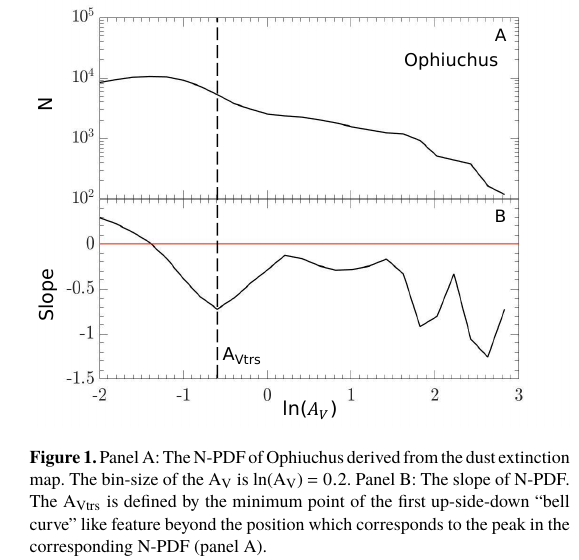MCF is the amount of cloud mass that is above a given dust extinction, $\tilde A_V$,

$\MCF(\hat A_V) = \mu m_HC\sum_{\pixel (A_V>\tilde A_V)} A_V \times A_{\pixel}$

where

• $\mu=1.37$ is the mean molecular weight
• $m_H$ is the mass of hydrogen
• $A_\pixel = [D(cm)\times R(rad)]^2$ is the area of one pixel
• $C=1.37\times 10^{21}\cm^{-2}\mag^{-1}$

The MCF slope is defined by

$\text{MCF slope} = \left\vert \frac{\log(M_{trs})-\log (0.1M_\trs)}{A_{V\trs}-A_{V0.1}}\right\vert = \frac{1}{\vert A_{V\trs} - A_{V0.1}}$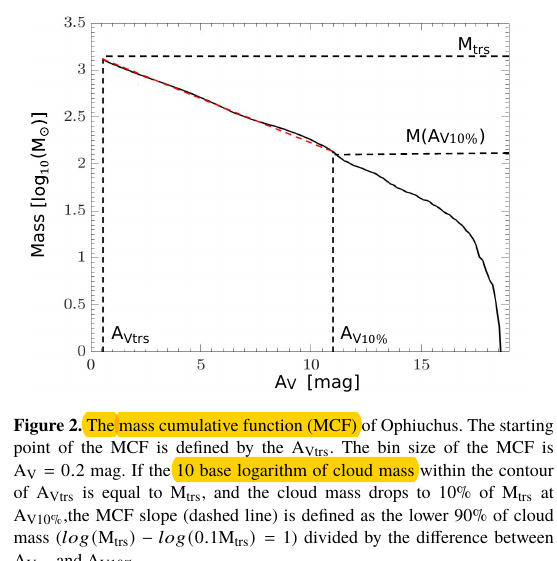### Results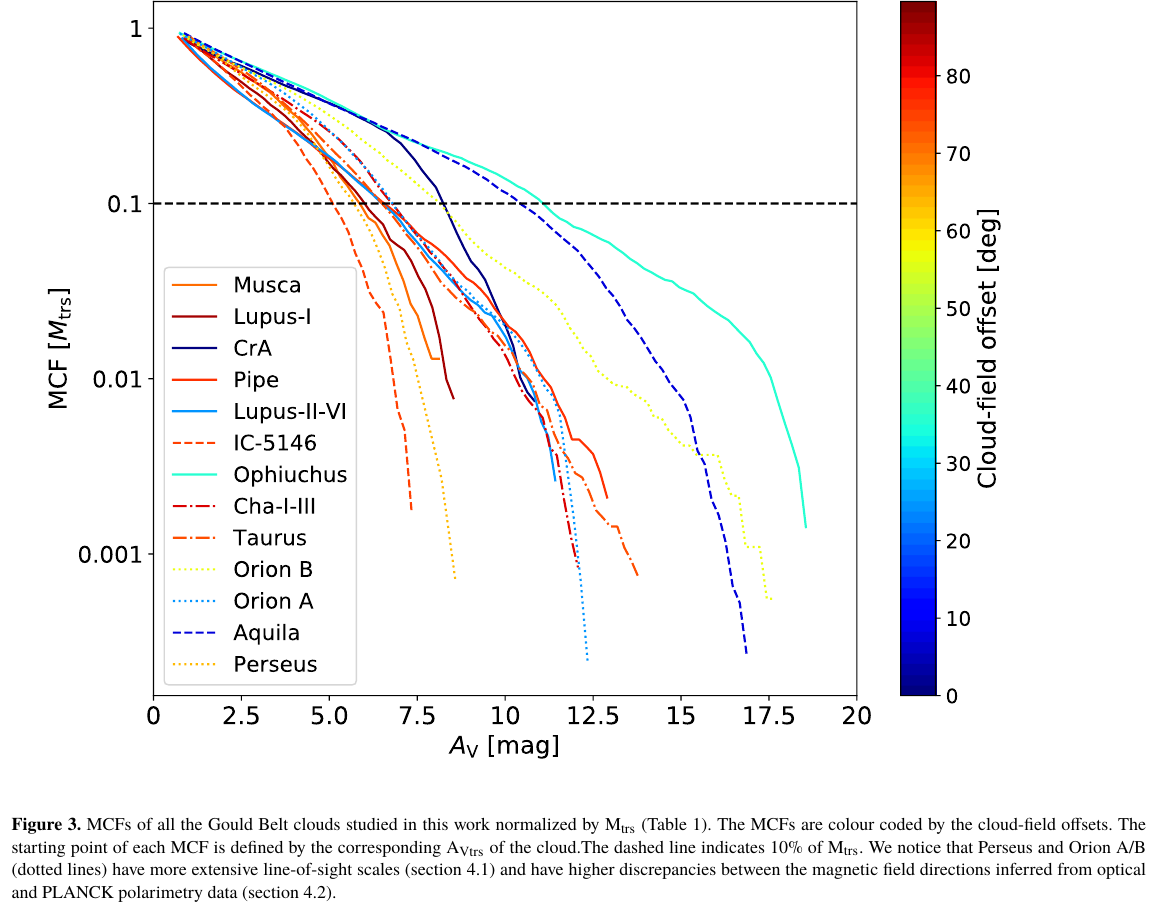• molecular clouds with larger cloud-field offsets show steeper slopes

study the degree of significance of the correction between MCF slopes and cloud-field offsets

$H_0: \mu_{\small} = \mu_{\large}\qquad H_1:\mu_\small > \mu_\large$

where $\mu_\small$ is the average MCF slope of the molecular clouds with cloud-field offsets < 45 degrees.

By the non-parametric permutation test, the resulting $p$-value is 0.01.

### MCF slope uncertainties

The potential uncertainties in the MCF slopes arising from

• extinction measurement uncertainty
• line of sight (LOS) contamination
• MCF bin width
• spatial resolution
• $A_{V\trs}$ uncertainty
• $A_V$ range adopted for the MCF slope measurements

Published in categories Note